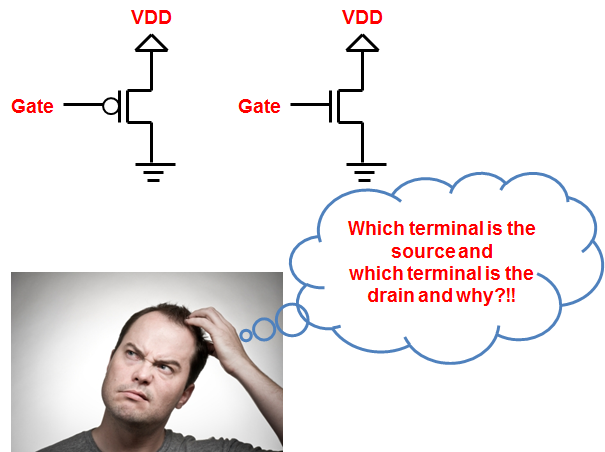﻿ Drain and the Source of MOS TransistorsBack

## Drain and the Source of MOS TransistorsWhile most of us are taught one basic rule in our introduction classes to the CMOS Transistor: The source of an NMOS is typically at the lowest potential while the source of a PMOS transistor is at the highest potential.

While this rule is correct, there got to be a plausible technical explanation behind this nomenclature. In this post, I'll discuss that explanation.

First of all one must appreciate the fact that unlike BJT, MOSFET is a symmetric structure. By this, I mean that any terminal can act as drain or source depending upon the voltage applied on that particular terminal. In NMOS: Source is the terminal which is at the lowest potential. In PMOS, Source is the terminal with the highest potential. Now, the question arrives why did we choose to call a particular terminal as source or drain. Read on.

In NMOS, the majority charge carriers are the electrons. If you've followed the physics behind the transistor operation, you'd know that current in an NMOS flows from Drain to Source. Alternatively, electrons flow from the source to drain. Source is therefore the "reservoir" or "source" of electrons which get "drained" at the Drain! That's where the terminology comes from. Therefore, the source of electrons in an NMOS has to be connected to the lowest potential, that being the VSS. And Drain to the higher potential, which could perhaps be VDD.

In PMOS, the majority charge carriers are the holes. Holes will flow from higher potential to a lower potential. Higher potential terminal would act as the "source" of holes. That would therefore be VDD. These holes would get "drained" at a lower potential, which could perhaps be VSS. Therefore, for PMOS, higher potential terminal would be the Source, and lower potential terminal would be the drain!